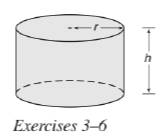Chapter 9.3, Problem 3EElementary Geometry For College St...

7th Edition
Alexander + 2 others
ISBN: 9781337614085

Solutions

Chapter
SectionElementary Geometry For College St...

7th Edition
Alexander + 2 others
ISBN: 9781337614085
Textbook Problem

For the right circular cylinder, r = 3.2 cm and h = 5.1 cm. Find the approximate volume of the cylinder.To determine

To calculate:

The approximate volume of the cylinder.

Explanation

Given:

For a right circular cylinder r=3.2cm and h=5.1cm.

Formula used:

The volume of the right circular cylinder is V=πr2h.

Calculation:

Substitute the known values in the formula,

V=πr2h=π(3

Still sussing out bartleby?

Check out a sample textbook solution.

See a sample solution

The Solution to Your Study Problems

Bartleby provides explanations to thousands of textbook problems written by our experts, many with advanced degrees!

Get Started

24. Find

Mathematical Applications for the Management, Life, and Social Sciences

In words, explain what is measured by SS, variance, and standard deviation.

Statistics for The Behavioral Sciences (MindTap Course List)

In Exercises 516, evaluate the given quantity. log4164

Finite Mathematics and Applied Calculus (MindTap Course List)